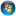# 一份还算可以的PHP面试题

<?
\$str1 = null;
\$str2 = false;
echo \$str1==\$str2 ? ‘相等’ : ‘不相等’;
\$str3 = ”;
\$str4 = 0;
echo \$str3==\$str4 ? ‘相等’ : ‘不相等’;

\$str5 = 0;
\$str6 = ‘0′;
echo \$str5===\$str6 ? ‘相等’ : ‘不相等’;
?>
2. 写出如下程序的输出结果
<?
\$a1 = null;
\$a2 = false;
\$a3 = 0;
\$a4 = ”;
\$a5 = ‘0′;
\$a6 = ‘null’;
\$a7 = array();
\$a8 = array(array());

echo empty(\$a1) ? ‘true’ : ‘false’;
echo empty(\$a2) ? ‘true’ : ‘false’;
echo empty(\$a3) ? ‘true’ : ‘false’;
echo empty(\$a4) ? ‘true’ : ‘false’;
echo empty(\$a5) ? ‘true’ : ‘false’;
echo empty(\$a6) ? ‘true’ : ‘false’;
echo empty(\$a7) ? ‘true’ : ‘false’;
echo empty(\$a8) ? ‘true’ : ‘false’;
?>

3. 写出如下程序的输出结果
<?
\$test = ‘aaaaaa’;
\$abc = & \$test;
unset(\$test);

echo \$abc;
?>

4. 写出如下程序的输出结果
<?\$count = 5;
function get_count(){
static \$count = 0;
return \$count++;
}

echo \$count;
++\$count;
echo get_count();
echo get_count();
?>

5. 写出如下程序的输出结果
<?
\$GLOBALS['var1'] = 5;
\$var2 = 1;
function get_value(){
global \$var2;
\$var1 = 0;
return \$var2++;
}
get_value();

echo \$var1;
echo \$var2;
?>

6. 写出如下程序的输出结果
<?
function get_arr(\$arr){
unset(\$arr);
}
\$arr1 = array(1, 2);
\$arr2 = array(1, 2);

get_arr(&\$arr1);
get_arr(\$arr2);

echo count(\$arr1);
echo count(\$arr2);
?>
7. 使用五种以上方式获取一个文件的扩展名

1. 使用PHP描述冒泡排序和快速排序算法，对象可以是一个数组

2. 使用PHP描述顺序查找和二分查找（也叫做折半查找）算法，顺序查找必须考虑效率，对象可以是一个有序数组

3. 写一个二维数组排序算法函数，能够具有通用性，可以调用php内置函数
【附答案】（以下答案不一定是最好的，只是一个简单的参考）

1. 相等 相等 不相等
2. true true true true true false true false
3. aaaaaa
4. 5 0 1
5. 5 2
6. 1 2
7. 使用五种以上方式获取一个文件的扩展名

function get_ext1(\$file_name){
return strrchr(\$file_name, ‘.’);
}
function get_ext2(\$file_name){
return substr(\$file_name, strrpos(\$file_name, ‘.’));
}
function get_ext3(\$file_name){
return array_pop(explode(‘.’, \$file_name));
}
function get_ext4(\$file_name){
\$p = pathinfo(\$file_name);
return \$p['extension'];
}
function get_ext5(\$file_name){
return strrev(substr(strrev(\$file_name), 0, strpos(strrev(\$file_name), ‘.’)));
}

1. 使用PHP描述冒泡排序和快速排序算法，对象可以是一个数组

//冒泡排序（数组排序）
function bubble_sort(\$array)
{
\$count = count(\$array);
if (\$count <= 0) return false;

for(\$i=0; \$i<\$count; \$i++){
for(\$j=\$count-1; \$j>\$i; \$j–){
if (\$array[\$j] < \$array[\$j-1]){
\$tmp = \$array[\$j];
\$array[\$j] = \$array[\$j-1];
\$array[\$j-1] = \$tmp;
}
}
}
return \$array;
}

//快速排序（数组排序）
function quick_sort(\$array) {
if (count(\$array) <= 1) return \$array;

\$key = \$array;
\$left_arr = array();
\$right_arr = array();

for (\$i=1; \$i<count(\$array); \$i++){
if (\$array[\$i] <= \$key)
\$left_arr[] = \$array[\$i];
else
\$right_arr[] = \$array[\$i];
}

\$left_arr = quick_sort(\$left_arr);
\$right_arr = quick_sort(\$right_arr);

return array_merge(\$left_arr, array(\$key), \$right_arr);
}
2. 使用PHP描述顺序查找和二分查找（也叫做折半查找）算法，顺序查找必须考虑效率，对象可以是一个有序数组

//二分查找（数组里查找某个元素）
function bin_sch(\$array, \$low, \$high, \$k){
if (\$low <= \$high){
\$mid = intval((\$low+\$high)/2);
if (\$array[\$mid] == \$k){
return \$mid;
}elseif (\$k < \$array[\$mid]){
return bin_sch(\$array, \$low, \$mid-1, \$k);
}else{
return bin_sch(\$array, \$mid+1, \$high, \$k);
}
}
return -1;
}

//顺序查找（数组里查找某个元素）
function seq_sch(\$array, \$n, \$k){
\$array[\$n] = \$k;
for(\$i=0; \$i<\$n; \$i++){
if(\$array[\$i]==\$k){
break;
}
}
if (\$i<\$n){
return \$i;
}else{
return -1;
}
}
3. 写一个二维数组排序算法函数，能够具有通用性，可以调用php内置函数

//二维数组排序， \$arr是数据，\$keys是排序的健值，\$order是排序规则，1是升序，0是降序
function array_sort(\$arr, \$keys, \$order=0) {
if (!is_array(\$arr)) {
return false;
}
\$keysvalue = array();
foreach(\$arr as \$key => \$val) {
\$keysvalue[\$key] = \$val[\$keys];
}
if(\$order == 0){
asort(\$keysvalue);
}else {
arsort(\$keysvalue);
}
reset(\$keysvalue);
foreach(\$keysvalue as \$key => \$vals) {
\$keysort[\$key] = \$key;
}
\$new_array = array();
foreach(\$keysort as \$key => \$val) {
\$new_array[\$key] = \$arr[\$val];
}
return \$new_array;
}

2007-06-25 10:36

* 1.在PHP中，当前脚本的名称（不包括路径和查询字符串）记录在预定义变量（1）中；而链接到当前页面的URL记录在预定义变量（2）中。
2.执行程序段<?php echo 8%(-2) ?>将输出（3）。
3.在HTTP 1.0中，状态码 401 的含义是（4）；如果返回“找不到文件”的提示，则可用 header 函数，其语句为（5）。
4.数组函数 arsort 的作用是（6）；语句 error_reporting(2047)的作用是（7）。
5.PEAR中的数据库连接字符串格式是（8）。
6.写出一个正则表达式，过虑网页上的所有JS/VBS脚本（即把script标记及其内容都去掉）：（9）。
7.以Apache模块的方式安装PHP，在文件http.conf中首先要用语句（10）动态装载PHP模块，

8.语句 include 和 require 都能把另外一个文件包含到当前文件中，它们的区别是（12）；为了避免多次包含同一文件，可以用语句（13）来代替它们。
9.类的属性可以序列化后保存到 session 中，从而以后可以恢复整个类，这要用到的函数是（14）。
10.一个函数的参数不能是对变量的引用，除非在php.ini中把（15）设为on.
11.SQL 中LEFT JOIN的含义是（16）。

tbl_score记录了学生（有的学生考试以后被开除了，没有其记录）的学号(ID)和考试成绩(score)以及考试科目(subject)，

12.在PHP中，heredoc是一种特殊的字符串，它的结束标志必须（18）。

13.写一个函数，能够遍历一个文件夹下的所有文件和子文件夹。
14.简述论坛中无限分类的实现原理。
15.设计一个网页，使得打开它时弹出一个全屏的窗口，该窗口中有一个文本框和一个按钮。用户在文本框中输入信息后点击按钮就可以把窗口关闭，而输入的信息却在主网页中显示。

//答案（填空）:

1. echo \$_SERVER['PHP_SELF']; echo \$_SERVER["HTTP_REFERER"];
2. 0
4. (6)对数组进行逆向排序并保持索引关系   (7)All errors and warnings
5. 没弄明白
6. /<script[^>].*?>.*?<\/script>/si

AddType application/x-httpd-php .php .php5 .php4 .php3 .phtml
8.(12) 发生异常时include产生警告require产生致命错误   (13) require_once()/include_once()
9. serialize() /unserialize()
10. allow_call_time_pass_reference
11. (16) 自然左外连接
(17) select name , count(score) as sum_score from tbl_user left join tbl_score on tbl_user.ID=tbl_score.ID   group by tbl_user.ID
12. 结束标识符所在的行不能包含任何其它字符除”;”

13.

/**
* 遍历目录，结果存入数组。支持php4及以上。php5以后可用scandir()函数代替while循环。
* @param string \$dir
* @return array
*/
function my_scandir(\$dir)
{
\$files = array();
if ( \$handle = opendir(\$dir) ) {
while ( (\$file = readdir(\$handle)) !== false ) {
if ( \$file != “..” && \$file != “.” ) {
if ( is_dir(\$dir . “/” . \$file) ) {
\$files[\$file] = rec_scandir(\$dir . “/” . \$file);
}else {
\$files[] = \$file;
}
}
}
closedir(\$handle);
return \$files;
}
}

 分享到：
• DH• 2011年08月16日

原来如此

• 匿名• 2011年08月16日

哈哈  我个人认为这些题都是很不错的面试题啊。
经验 经验 经验啊～
不在乎难度，更追求技巧。 不会是阁下自己出的吧？

有着 ”整蛊专家“，“破坏之王” の称 的 チェン教師…

• Clove• 2011年08月16日

小泉,就算你匿名了,我也知道是你
没事给俺点广告去,话说广告账户都快被取消了…

• ms_compound• 2011年08月20日

杯具啊….，Sir 。 这次真不是….
ms_compound 这名字全世界就我自己用
虽然您可能想不起我是谁，但是您的课，是我最喜欢上的.
其实您是我最佩服的人，勤恳，老牛，低调。

被爱情埋葬之后的男银就是不一样啊。。

• Clove• 2011年08月20日

我去,我还以为是小泉纯一郎呢

• DH• 2011年08月16日

很多都不会，悲剧啊

• Clove• 2011年08月16日

面试就是考官当面显摆一下，让你以为自己是陀屎
实际工作了才知道，大家一样屎

1. 没有通告